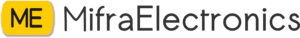Sale!

# 150K ohm Resistors

20.00

Availability: 50 in stock

SKU: MT0831 Category:

## What is Resistor?

Resistor is defined as

A passive electrical component with two terminals that are used for either limiting or regulating the flow of electric current in electrical circuits.

The main purpose of resistor is to reduce the current flow and to lower the voltage in any particular portion of the circuit. It is made of copper wires which is coiled around a ceramic rod and the outer part of the resistor is coated with an insulating paint.

### What is the SI Unit of Resistor?

The SI unit of resistor is Ohm.

Each resistor has one connection and two terminals. We will look at the three types of symbols that are used to represent the resistor.The terminals of the resistor are each of the lines extending from the squiggle (or rectangle). Those are what connect to the rest of the circuit. The resistor circuit symbols are usually enhanced with both a resistance value and a name. The value, displayed in ohms, is obviously critical for both evaluating and actually constructing the circuit.

## What is Color Coding of Resistors?

Resistors may not display the value outside but resistor color pattern through their resistance can be calculated. PTH (plated-through-hole) resistors use a color-coding system (which really adds some flair to circuits), and SMD (surface-mount-device)resistors have their own value-marking system.

Following is a table with color code of resistors:

 Color Color code Black 0 Brown 1 Red 2 Orange 3 Yellow 4 Green 5 Blue 6 Violet 7 Grey 8 White 9

### What is Tolerance in Resistors?

Following is a table with tolerance of resistor:

 Colour Tolerance Brown ±1% Red ±2% Gold ±5% Silver ±10%

## Resistors in Series

Resistors are said to be in series when the current flowing through all the resistors is the same. These resistors are connected from head to tail in series. The overall resistance of the circuit is equal to the sum of individual resistance values.### Resistors in Series Formula

 Rtotal = R1 + R2 + R3 +……+Rn

Where,

• Rtotal is the sum of all the individual resistances

## Resistors in Parallel

Resistors are said to be in parallel when the terminals of resistors are connected to the same two nodes. Resistors in parallel share the same voltage at their terminals.

### Resistors in Parallel Formula

 $$\frac{1}{R_{total}}=\frac{1}{R_{1}}+\frac{1}{R_{2}}+…..+\frac{1}{R_{n}}$$

Where,

• $$\frac{1}{R_{total}}$$ is the sum of all the individual resistances

## Applications of Resistor

Following are the applications of resistors:

• Wire wound resistors find application where balanced current control, high sensitivity, and accurate measurement are required like in shunt with ampere meter.
• Photo resistors find application in flame detectors, burglar alarm, in photographic devices, etc.
• Resistors are used for controlling temperature and voltmeter.
• Resistors are used in digital multi-meter, amplifiers, telecommunication, and oscillators.
• They are also used in modulators, demodulators, and transmitters.keep in touch with our social media links as mentioned below
Mifratech Note : Find the best solution for electronics components and technical projects ideas